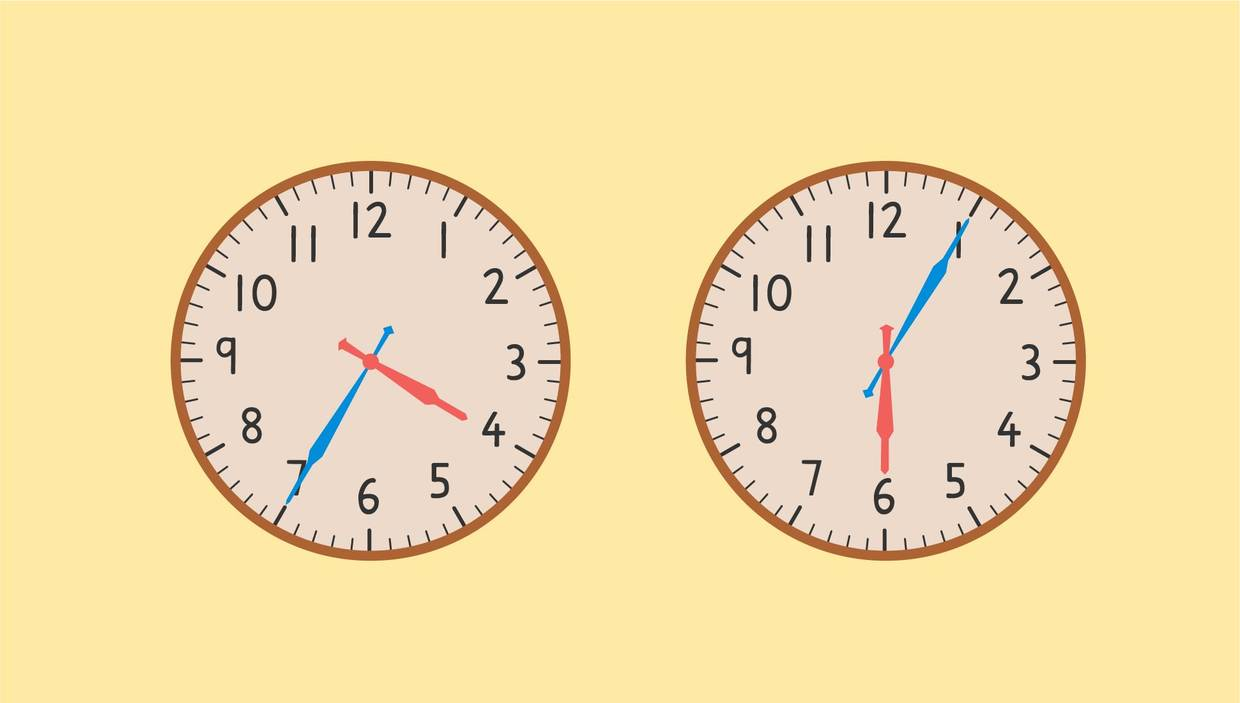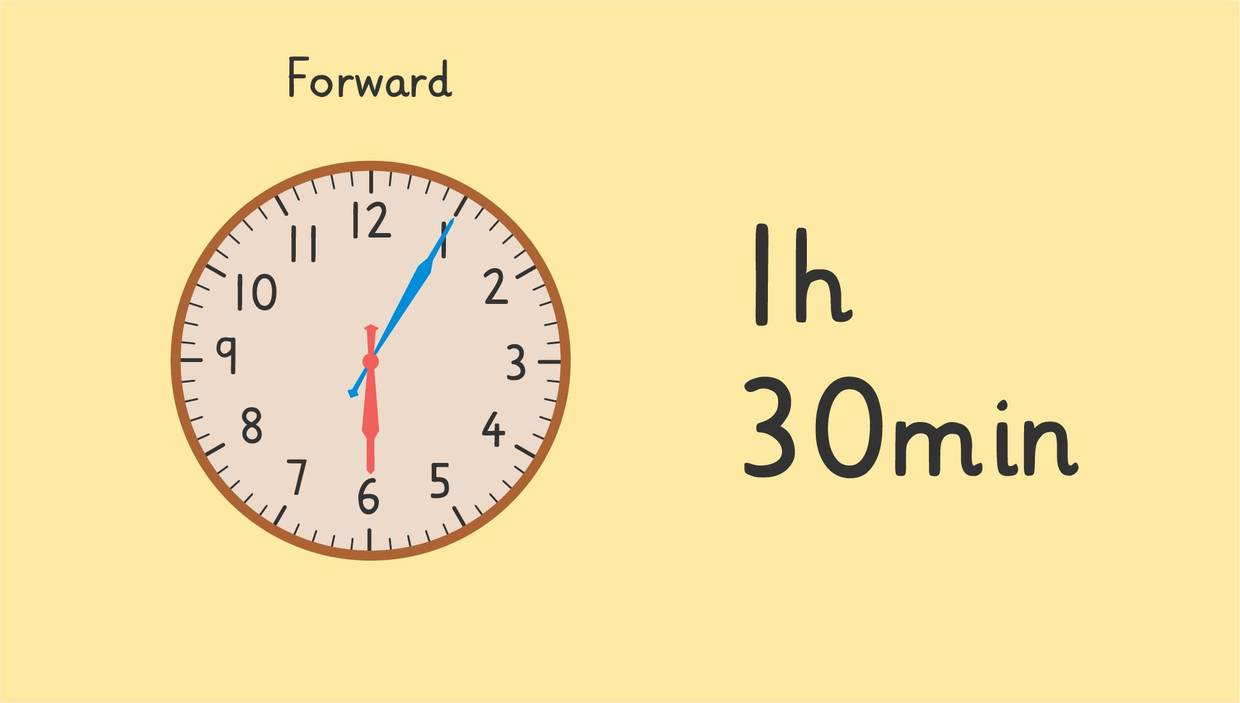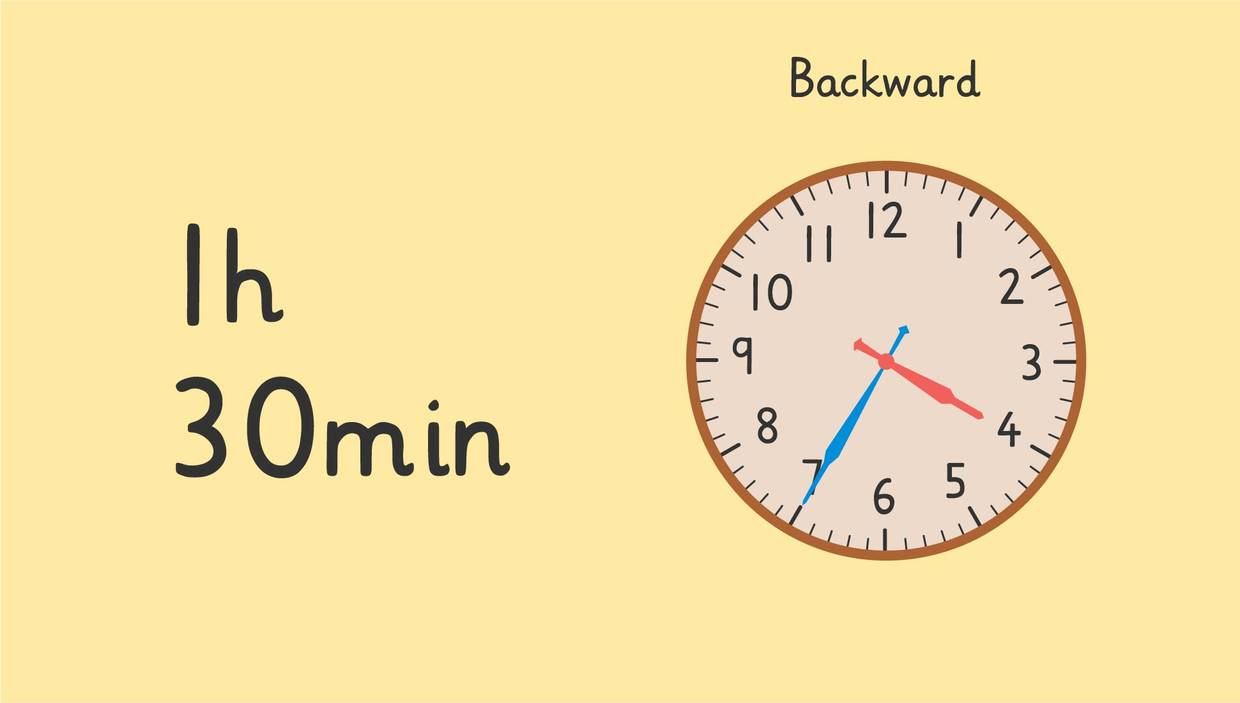# Time IntervalsRate this video

Ø 5.0 / 3 ratings

The authorTeam Digital

## DescriptionTime Intervals

### Contents

• What is a time interval?
• ### What is a time interval?

You might ask yourself, what does time interval mean? The definition for time interval is how long an activity takes from beginning to end.

#### How to find time interval and Time Interval Calculation

We can use a clock to calculate the interval of time it takes to complete a task. You can skip count forward from the start time to the end time to find the time interval, or you can skip count backward from the end time to the start time to find the time interval. When you calculate the interval of time, it can help you find which interval of time is the longest or shortest. Let’s take a look at a time interval example.

#### Time Interval Example

Zuri is baking some desserts for a surprise party for Freddie and is trying to calculate the interval time it takes to bake each item. Remember, the time interval meaning is how long an activity takes from beginning to end.

She put the cake in the oven at four thirty-five and took them out at six o’five. The first step in a time interval calculation is to find the start time and the end time on the clock.The second step is to skip count forward from the start time to the end time to find the time interval, or you can skip count backward from the end time to the start time to find the time interval.

If we count forward from the start time to the end time, we can skip count by one hour first, which brings us to five thirty-five. From there we can skip count by tens until we reach six o’five. It takes three tens to reach four thirty-five so we know the time interval it takes to bake the cake is one hour and thirty minutes.If we skip count backward from the end time to the start time, we can skip count by one hour first which brings us to five o’five. From there we can skip count by tens until we reach four thirty-five. It takes three tens to reach four thirty-five so we know the time interval it takes to bake the cake is one hour and thirty minutes.As you can see, there are two different ways to do a time interval calculation but we still get the same time interval.

#### How to find time Interval and Time Interval Calculation Summary

Remember, the time interval definition is how long an activity takes from start to finish. We can calculate the time interval using two easy steps:

• Step 1: Find the start time and the end time on the clock
• Step 2: Skip count forward from the start time to the end time to find the time interval, or you can skip count backward from the end time to the start time

Have you practiced yet? On this website, you can also find time interval worksheets and exercises.

### TranscriptTime Intervals

Zuri is preparing treats for Freddie's surprise birthday party. She's baking cupcakes, cookies, and a cake. A time interval is how long an activity takes from beginning to end. We can use the clock to calculate Time intervals. What are some ways we can skip count to calculate the minutes? We can calculate by skip counting five minutes and ten minutes. You can skip count forward from the start time to the end time or you can skip count backward from the end time to the start time. Let's see how long it took Zuri to bake the cookies! The first step is to find the start time and the end time on the clock. They started baking at two twenty and finished at three oh five. The second step is to count forward from the start time to calculate the time interval. Here we can skip count by fives. Five, ten, fifteen, twenty, twenty-five, thirty, thirty-five, forty, forty-five. How long did the cookies take to bake? The time interval it took for Zuri to bake the cookies is forty-five minutes. Now let's see how long it took for Zuri to bake the cupcakes. The first step is to find the start time and the end time on the clock. Zuri put them in the oven at three fifteen and took them out at four twenty-five. Can you find the start time and the end time on the clock? They started here at three fifteen and ended here at four twenty-five. This time we will count backward from the end time to calculate the time interval. Since we know from four twenty-five to three fifteen is more than one hour, we can skip count backward by one hour first. That means the hour hand moves from the four to the three. That brings us to three twenty-five, can you skip count backward by fives until we reach three fifteen? Five, ten! How long did it take for the cupcakes to bake? The time interval it took to bake the cupcakes is one hour and ten minutes. Now let's see how long it took for Zuri to bake the cake. Zuri put the cake in the oven at four thirty-five. She took the cake out at six oh five. How long did it take to bake the cake? Remember, the first step is to find the start time and the end time on the clock. The second step is to count forward from the start time or backward from the end time to calculate the time interval. This time you can choose to skip count forward or backward. If you decided to count forward from the start time, you could have skip counted by one hour. That means the hour hand moves from the four to the five. That brings you to five thirty-five. From there, you could have counted by tens until you reached six oh five. Ten, twenty, thirty.The time interval it took to bake the cake is one hour and thirty minutes. Let's see if we get the same time interval when we count backward. If you decided to count backward from the end time, you could have skip counted by one hour. That means the hour hand moves from the six to the five. That brings you to five oh five. From there, you could have counted by tens until you reached four thirty-five. Ten, twenty, thirty. Counting backward, we also find the time interval is one hour and thirty minutes. Remember, when you are trying to identify the time interval, you are trying to see how long an activity takes from beginning to end. The first step is to find the start time and the end time. The second step is to skip count forward from the start time(...)or you can skip count backward from the end time. Oh! It looks like it's time to get ready to surprise Freddie...shhh! "Quick, everybody hide!" "Surprise!"

## Time Intervals Exercise

Would you like to practice what you’ve just learned? Practice problems for this video Time Intervals help you practice and recap your knowledge.
• ### Choose the set of clocks that match the time interval.

Hints

Skip count forward from the start time or skip count backward from the end time.

Remember to skip count by 5's between each number.

Solution

Counting Forward:

• Starting at 3:15 we can count forward 1 hour.
• That means the hour hand moves from 3 to 4, which brings us to 4:15.
• After skip counting forward to reach 4:25, we get 10 minutes.
• The time interval it took was 1 hour and 10 minutes.
Counting Backward:
• Starting at 4:25 we can count backward 1 hour.
• That means the hour hand moves from 4 to 3, which brings us to 3:25.
• After skip counting backward to reach 3:15, we get 10 minutes.
• The time interval it took was 1 hour and 10 minutes.

• ### Match the time interval to the clocks.

Hints

Skip count forward from the start time or skip count backward from the end time.

Has more than an hour passed?

Remember to skip count by 5's between each number.

Solution

Counting backward:
For the first set of clocks:

• Starting at 1:35 we can skip count backward to reach 12:50, which is 45 minutes.
• The time interval it took was 45 minutes.
_____________________________________________________________________________________ For the other clocks counting backward:
For the second set of clocks:
• Starting at 8:30 we can count backward 1 hour. That means the hour hand moves from 8 to 7, which brings us to 7:30.
• After skip counting backward to reach 7:25, we get 5 minutes.
• The time interval it took was 1 hour and 5 minutes.
For the third set of clocks:
• Starting at 3:40 we can skip count backward to reach 2:45, which is 55 minutes.
• The time interval it took was 55 minutes.
For the last set of clocks:
• Starting at 7:45 we can count backward 1 hour. That means the hour hand moves from 7 to 6, which brings us to 6:45.
• After skip counting backward to reach 6:15, we get 30 minutes.
• The time interval it took was 1 hour and 30 minutes.

• ### How long did each task take?

Hints

Skip count forward from the start time or skip count backward from the end time.

Remember to skip count by 5's between each number.

Solution

Counting forward:
For the first set of clocks:

• Starting at 10:15 we can skip count forward to reach 11:05, which is 50 minutes.
• The time interval it took was 50 minutes.
_____________________________________________________________________________________ For the other clocks counting forward:
For the second set of clocks:
• Starting at 6:25 we can count forward 1 hour. That means the hour hand moves from 6 to 7, which brings us to 7:25.
• After skip counting forward to reach 7:50, we get 25 minutes.
• The time interval it took was 1 hour and 25 minutes.
For the third set of clocks:
• Starting at 3:40 we can count forward 1 hour. That means the hour hand moves from 3 to 4, which brings us to 4:40.
• After skip counting forward to reach 4:55, we get 15 minutes.
• The time interval it took was 1 hour and 15 minutes.
For the last set of clocks:
• Starting at 12:05 we can skip count forward to reach 12:50, which is 45 minutes.
• The time interval it took was 45 minutes.

• ### What's the time interval?

Hints

Try solving one of two ways:

• Skip count the time interval backward starting at the end time
• Skip count forward from the start time and figure out which interval and end time matches

Has more than an hour passed?

Remember to skip count by 5's between each number.

Solution

Counting backward:
Starting at 4:05 and the time interval 1 hour and 30 minutes:

• We can count backward 1 hour. That means the hour hand moves from 4 to 3, which brings us to 3:05.
• After skip counting backward 30 minutes, we stop at 2:35.
_____________________________________________________________________________________ For the other clocks counting backward:
Starting at 3:05 and the time interval 45 minutes:
• We can skip count backward 45 minutes, which brings us to 2:20.
Starting at 3:40 and the time interval 1 hour and 25 minutes:
• We can count backward 1 hour. That means the hour hand moves from 3 to 2, which brings us to 2:40.
• After skip counting backward 25 minutes, we stop at 2:15.
Starting at 3:20 and the time interval 35 minutes:
• We can skip count backward 35 minutes, which brings us to 2:45.

• ### How long did it take Zuri to bake the cupcakes?

Hints

Skip count forward from the start time or skip count backward from the end time.

Has more than an hour passed?

Remember to skip count by 5's between each number.

Solution

Counting Forward:

• Starting at 3:15 we can count forward 1 hour.
• That means the hour hand moves from 3 to 4, which brings us to 4:15.
• After skip counting forward to reach 4:25, we get 10 minutes.
• The time interval it took was 1 hour and 10 minutes.
Counting Backward:
• Starting at 4:25 we can count backward 1 hour.
• That means the hour hand moves from 4 to 3, which brings us to 3:25.
• After skip counting backward to reach 3:15, we get 10 minutes.
• The time interval it took was 1 hour and 10 minutes.

• ### Which time intervals are wrong?

Hints

Skip count forward from the start time or skip count backward from the end time.

Has more than an hour passed?

Remember to skip count by 5's between each number.

Two time intervals are wrong.

Solution

The second and last time intervals are correct.
The first time interval is 35 minutes, not 45 minutes.

• Starting at 6:25 we can skip count backward to reach 5:50, which would be 35 minutes.
• The time interval it took was 35 minutes.
The third time interval is 1 hour & 40 minutes, not 2 hours & 30 minutes.
• Starting at 5:15 we can count backward 1 hour. That means the hour hand moves from 5 to 4, which brings us to 4:15.
• After skip counting backward to reach 3:35, we get 40 minutes.
• The time interval it took was 1 hour and 40 minutes.